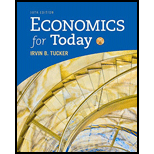# The equation for a budget line for goods X and Y , with P x being the price of X , P y being the price of Y , and B being the budget, can be written as a. P x X + P y Y = B . b. P x X + P y Y = 1/ B . c. P x X = B + P y Y . d. P x X / P y Y = 1 – B .### Economics For Today

10th Edition
Tucker
Publisher: Cengage Learning
ISBN: 9781337613040### Economics For Today

10th Edition
Tucker
Publisher: Cengage Learning
ISBN: 9781337613040

#### Solutions

Chapter
Section
Chapter 6.A, Problem 8SQ
Textbook Problem

## Expert Solution

### Want to see the full answer?

Check out a sample textbook solution.See solution

### Want to see this answer and more?

Experts are waiting 24/7 to provide step-by-step solutions in as fast as 30 minutes!*

See Solution

*Response times vary by subject and question complexity. Median response time is 34 minutes and may be longer for new subjects.